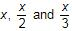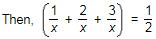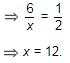Courses

# Time And Work MCQ 1

## 20 Questions MCQ Test Quantitative Techniques for CLAT | Time And Work MCQ 1

Description
This mock test of Time And Work MCQ 1 for Quant helps you for every Quant entrance exam. This contains 20 Multiple Choice Questions for Quant Time And Work MCQ 1 (mcq) to study with solutions a complete question bank. The solved questions answers in this Time And Work MCQ 1 quiz give you a good mix of easy questions and tough questions. Quant students definitely take this Time And Work MCQ 1 exercise for a better result in the exam. You can find other Time And Work MCQ 1 extra questions, long questions & short questions for Quant on EduRev as well by searching above.
QUESTION: 1

### A piece of work can be done by 6 men and 5 women in 6 days or 3 men and 4 women in 10 days. It can be done by 9 men and 15 women in how many days ?

Solution:

To calculate the answer we need to get 1 man per day work and 1 woman per day work.
Let 1 man 1 day work =x
and 1 woman 1 days work = y.
=> 6x+5y = 1/6
and 3x+4y = 1/10
On solving, we get x = 1/54 and y = 1/90
(9 men + 15 women)'s 1 days work =
(9/54) + (15/90) = 1/3
9 men and 15 women will finish the work in 3 days

QUESTION: 2

### A works twice as fast as B. if both of them can together finish a work in 12 days, B alone can do it in.

Solution: Let time taken by b to do the work=2t
:a= t
1 day work of a and b = 1/2t+1/t=1+2/2t
=3/2t_(1)
1day work of a and b =1/12_(2)
1/12=3/2t
t=18
b can do that work alone =18×2=36
QUESTION: 3

### A alone can complete a work in 12 days, while A and B together can complete the same work in 8 days. The number of days that B will take to complete the work alone is

Solution:
QUESTION: 4

A work could be completed in 100 days. Due to the absence of 10 workers it was completed in 110 days. The original number of workers was

Solution:
QUESTION: 5

A can do a job in 15 days B in 10 days and C in 30 days. If A is helped by B and C on every third day, the job will be completed in

Solution:
QUESTION: 6

Certain number of men can complete a job in 30 days. If there were 5 more men, it could be completed in 10 days less. How many men were there in the beginning?

Solution:
QUESTION: 7

A man is paid Rs. 20 for each day he works and loses Rs. 3 for each day he is idle. At the end of 60 days he gets Rs. 280. Then he was idle for

Solution:

Suppose the worker remained idle for x days . Then , he worked for (60−x) days.

∴        20(60−x)−3x=280
⇔     1200-23x=280
⇔     23x=920
⇔       x=40

QUESTION: 8

A takes double as much time as B or thrice as much time as C to finish a piece of work. Working together they can finish the work in 2 days. B alone can do the workin -

Solution:
QUESTION: 9

4 men and 6 women can complete a work in 8 days while 3 men and 7 women can complete it in 10 days. In how many days will 10 women complete the work.

Solution:
QUESTION: 10

72 men can build a wall 280 m long in 21 days. How many men will take 18 days to build a similar type of a wall of length 100 m.

Solution:
QUESTION: 11

Prem and Sham can do a job together in 7 days. Sham is 1¾  times more efficient than Prem. Same job can be done by sham alone in

Solution:
QUESTION: 12

If 3 men or 4 women can plough a field in 43 days, how long will 7 men and 5 women take to plough it.

Solution:
3 men or 4 women can plough the field in 43 days.

3 men = 4 women.

1 man = 4/3 women.

7 man = 28/3 women.

7 men and 5 women = 5 + (28/3) = 43/3 women.

4 women can plough the field in 43 days.

So,

1 woman can plough in = 43 *4 days.

Hence,

43/3 women can plough = (43*4 *3) /43 = 12 days.
QUESTION: 13

8 men can do a work in 12 days. After 6 days of work four more men were employed. In how many days would the remaining work be done.

Solution:
QUESTION: 14

A can finish a work in 24 days, B in 9 days and C in 12 days. B and C start the work but left after 3 days. The remaining work will be done by A in

Solution:
QUESTION: 15

A can do a work in the same time as B and C together can do it. If A and B together can do it in 10 days and C alone in 50 days, then B alone can do the work in

Solution:
QUESTION: 16

A and B can do a work in 12 days, B and C in 15 days, C and A in 20 days. A alone can do the work in

Solution:
QUESTION: 17

If 12 men and 16 boys can do a piece work in 5 days, 13 men and 24 boys can do it in four days then the ratio of the work done daily by a man and a boy is?

Solution:
QUESTION: 18

A and B can weave a carpet in 10 days and 15 days respectively. They begin to work together but B leaves after 2 days. In what time will A complete the remaining work.

Solution:
QUESTION: 19

Ram can do ¼ of the work in 3 days and Sham can do 1/6 of the same work in 4 days. How much will Ram get if both work together and are paid Rs. 180 in all.

Solution:
QUESTION: 20

A takes twice as much time as B or thrice as much time as C to finish a piece of work. Working together, they can finish the work in 2 days. B can do the work alone in:

Solution:

Suppose A, B and C takedays respectively to finish work.So, B takes (12/2) = 6 days to finish the work.